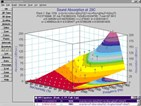# Automated Surface Fitting Software: TableCurve 3D®Automated Surface Fitting Software: TableCurve 3D® surface fitting software gives scientists and engineers the power to find the ideal model for even the most complex data, including equations that might never have been considered. TableCurve 3D's built-in equation set includes a wide array of linear and nonlinear models for any application.

Product Brochure: TableCurve 3D®: Automated Surface Fitting Software
30-Day Trial Software: TableCurve 3D®: Automated Surface Fitting Software

Find Optimum Equations to Describe Empirical Data

Automated Surface Fitting Software: TableCurve 3D® surface fitting software gives scientists and engineers the power to find the ideal model for even the most complex data, including equations that might never have been considered. TableCurve 3D's built-in equation set includes a wide array of linear and nonlinear models for any application.

• Linear equations
• Polynominal and rational functions
• Logrithmic and exponential functions
• Nonlinear peak functions
• Nonlinear transition functions
• Nonlinear exponential and power equations
• User-defined functions (up to 15)